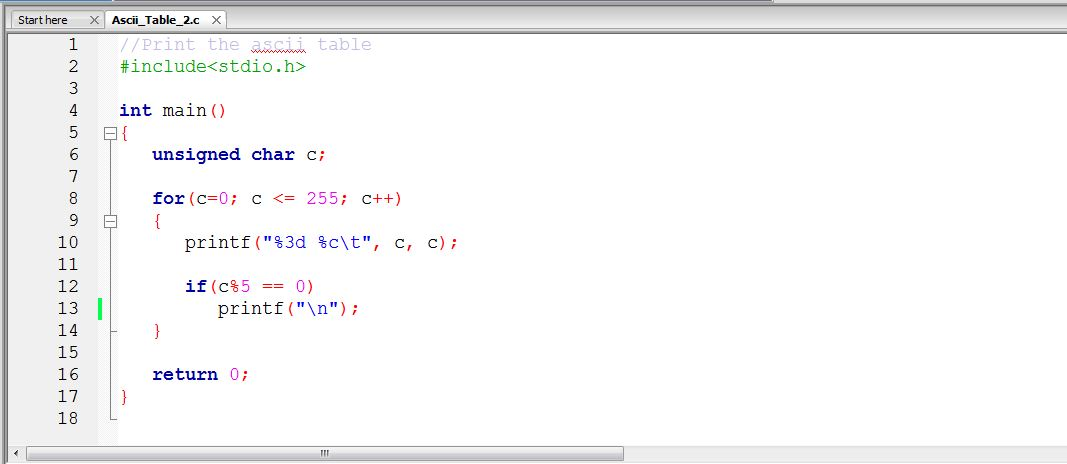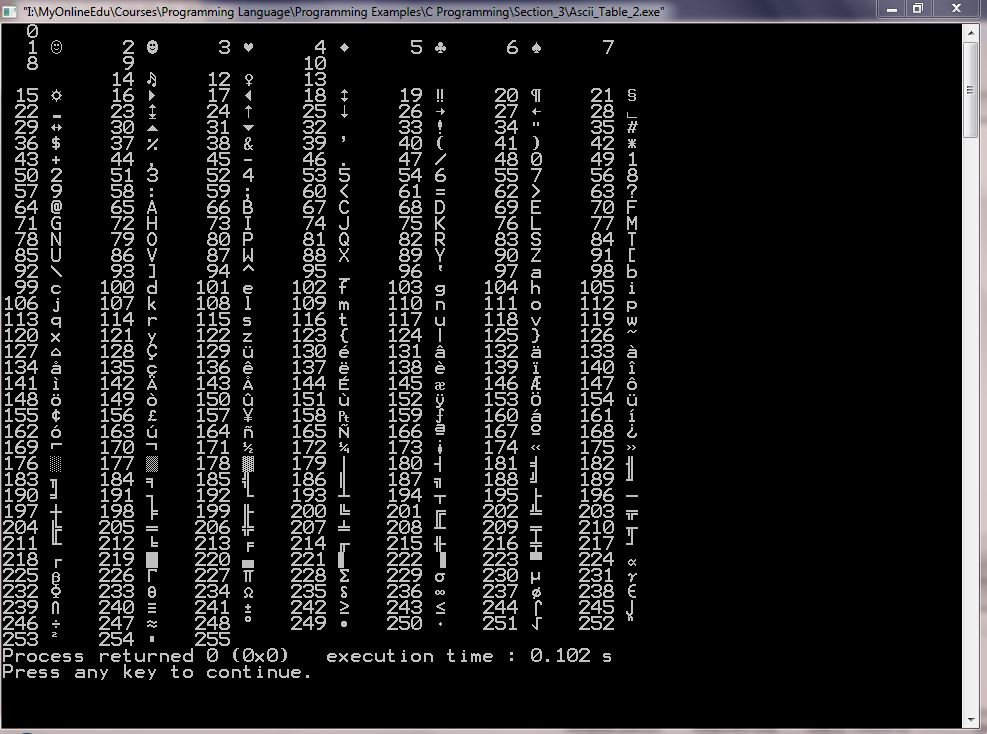# C Programming: Can you guess the output? Printing ASCII Table.Have you wondered why the above program results in infinite loop? Apparently there is no reason to be! It is a very simple program to print the ASCII table and we know that the ASCII value of characters range from 0 to 255 and it is prominent that the loop is going from 0 to 255. So why the infinite loop?

In this article, we will learn the reason but before that notice the below variable declaration.

``unsigned char c;``

Notice the data type is char but unsigned. The reason is simple as an char variable can store data from -128 to +127. Since we need to print the whole ASCII range from 0 to 255, we have to make it unsigned. This is making it possible to store values from 0 to 255 in variable c. But still the question remains why does it result in infinite loop? Before giving the answer. lets take a look at the correct program first.

//Print the ascii table

``````#include<stdio.h>
int main()
{
unsigned char c;
for(c=0; c <= 255; c++)
{
printf("= %c\t", c, c);

if(c%5 == 0)
printf("\n");
if(c == 255)
break;
}
return 0;
}``````

Notice the condition which is causing to stop the loop being infinite.

``````if(c == 255)
break;``````

This condition is necessary when the value of variable c is 255, and gets incremented with the expression c++, the value becomes 256. But the variable c which is unsigned character data type, can not store the the value 256 thus it makes it the start range value which is zero (0). Thus the condition evaluates to true always resulting in infinite loop.

The if condition is stopping the value in the variable c to become 256 and thus coming out of the loop.

Watch the video tutorial to know in details.

The output of the ASCII table is shown below (I have put the table in 7 columns to display in one screenshot):